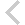FICO Xpress Optimization Examples Repository
 FICO Optimization Community FICO Xpress Optimization HomeFolio - Embedding examples from 'Getting started'

Description
Simple embedding tasks for a portfolio optimization problem:
• executing a Mosel model (folioexec.java)
• parameterized model execution (folioparam.java)
• exporting a matrix (foliomat.java)
• accessing model results (folioobj.java)
• data exchange in memory (runfolio.java,runfoliob.java,runfoliobd.java)
• retrieving solution output from Optimizer callbacks in foliocbio.mos during optimization (runfoliocbio.java)

Source Files

Data Files

foliocbio.mos

(!******************************************************
Mosel Example Problems
======================

file foliocbio.mos

Modeling a MIP problem
to perform portfolio optimization.

Same model as in foliomip3.mos.
-- Defining an integer solution callback
to write out solution information to external program --

*** This model cannot be run with a Community Licence
for the provided data instance ***

(c) 2011 Fair Isaac Corporation
author: S.Heipcke, July 2011, rev. Mar. 2022
*******************************************************!)

model "Portfolio optimization with MIP"
uses "mmxprs"
uses "mmjobs"

parameters
MAXRISK = 1/3                     ! Max. investment into high-risk values
MINREG = 0.2                      ! Min. investment per geogr. region
MAXREG = 0.5                      ! Max. investment per geogr. region
MAXSEC = 0.25                     ! Max. investment per ind. sector
MAXVAL = 0.2                      ! Max. investment per share
MINVAL = 0.1                      ! Min. investment per share
MAXNUM = 15                       ! Max. number of different assets
DATAFILE = "folio250.dat"         ! File with problem data
OUTPUTFILE = "sol10out.dat"       ! File for solution output
FRACSOL = "FRAC"                  ! Locations for solution output
NUMSHARES = "NUMSHARES"
RETSOL = "RETSOL"
SOLCOUNT = "SOLCOUNT"
end-parameters

forward procedure printsol

declarations
SHARES,S: set of string            ! Set of shares
RISK: set of string                ! Set of high-risk values among shares
REGIONS: set of string             ! Geographical regions
TYPES: set of string               ! Share types (ind. sectors)
LOC: array(REGIONS) of set of string ! Sets of shares per geogr. region
RET: array(SHARES) of real         ! Estimated return in investment
SEC: array(TYPES) of set of string ! Sets of shares per industry sector
end-declarations

initializations from DATAFILE
RISK RET LOC SEC
end-initializations

declarations
frac: array(SHARES) of mpvar      ! Fraction of capital used per share
buy: array(SHARES) of mpvar       ! 1 if asset is in portfolio, 0 otherwise
end-declarations

! Objective: total return
Return:= sum(s in SHARES) RET(s)*frac(s)

! Limit the percentage of high-risk values
sum(s in RISK) frac(s) <= MAXRISK

! Limits on geographical distribution
forall(r in REGIONS) do
sum(s in LOC(r)) frac(s) >= MINREG
sum(s in LOC(r)) frac(s) <= MAXREG
end-do

! Diversification across industry sectors
forall(t in TYPES) sum(s in SEC(t)) frac(s) <= MAXSEC

! Spend all the capital
sum(s in SHARES) frac(s) = 1

! Upper bounds on the investment per share
forall(s in SHARES) frac(s) <= MAXVAL

! Limit the total number of assets
sum(s in SHARES) buy(s) <= MAXNUM

forall(s in SHARES) do
buy(s) is_binary                  ! Turn variables into binaries
end-do

! Display Optimizer log
setparam("XPRS_verbose", true)

! Adapt Mosel comparison tolerance to Optimizer feasibility tolerance
setparam("zerotol", getparam("XPRS_feastol")/10)

! Set a MIP solution callback
setcallback(XPRS_CB_INTSOL, ->printsol)

! Solve the problem
maximize(Return)

if getprobstat <> XPRS_OPT then exit(1); end-if

!******** Solution output********

!**** Auxiliary function creating an array of solution values ****
function getvalues(v: array(SHARES) of mpvar): dynamic array(S) of real
forall(s in SHARES | v(s).sol<>0) returned(s):= v(s).sol
end-function

!**** Definition of the MIP solution callback function ****
procedure printsol
initializations to OUTPUTFILE
evaluation of getparam("XPRS_MIPSOLS") as SOLCOUNT
evaluation of sum(s in SHARES | buy(s).sol<>0) 1 as NUMSHARES
evaluation of getsol(Return) as RETSOL
evaluation of getvalues(frac) as FRACSOL